Acticles

DENSITY OF HYDROCARBONS (SPECIAL CASE)

Gas real (Pressure > 3 atm), you know the formula of density is :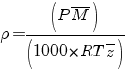P : atm R : 0.082 T : °K M :gr

However, if you want to estimate the density of mixture, it is possible you would introduce some correction.

When you have in the mixture, vapor of water, H2, O2, N2 etc, you need to considerate the hydrocarbons below a pressure equal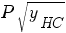instead of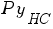. This change affected only the compute of compressible factor. (yHC is fraction molar of gas).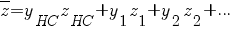where        z is the average compressible factor

zHC is the average compressible factor below a pressure equalz1, z2 is the compressible factor of compound 1, 2

y is the fraction molar of each compound.

Compute zHC : (this is the only change)

In order to computer it you need to have value :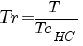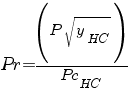Tr : reduce temperature Pr : reduce pressure TcHC critical or seudo-critical temperature PcHC critical or seudo-critical pressure yHC fraction molar of hydrocarbon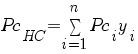PcHC critical or seudo-critical pressure Pci critical pressure of each hydrocarbons compound yi is the fracction molar of each hydrocarbons compound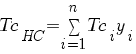TcHC critical or seudo-critical temperature Tci critical temperature of each hydrocarbons compound yi is the fracction molar of each hydrocarbons compound